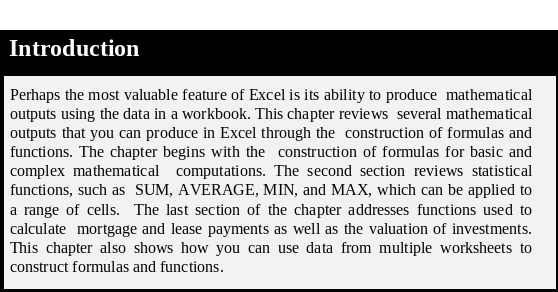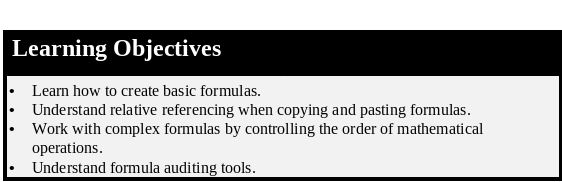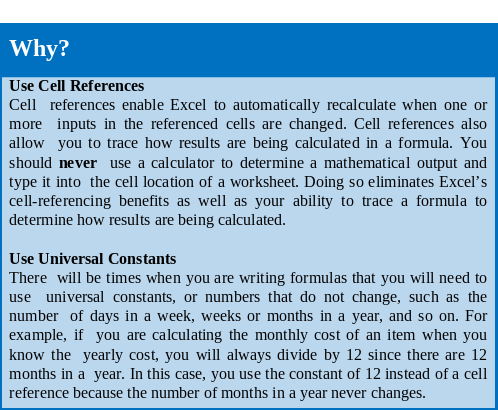### 1. FormulasThis section reviews the fundamental skills for entering formulas into an Excel worksheet. The example used for this chapter is the construction of a personal budget. Most financial advisors recommend that all households construct and maintain a personal budget to achieve and maintain strong financial health. Organizing and maintaining a personal budget is a skill you can practice at any point in your life. Whether you are managing your expenses during college or maintaining the finances of a family of four, a personal budget can be a vital tool when making financial decisions. Excel can make managing your money a fun and rewarding exercise.

## Open the Data File

1. Open the Data file named CH2 Data and use the File/Save As command to save it with the new name CH2 Personal Budget.

Figure 2.1 shows the completed workbook that will be demonstrated in this chapter. Notice that this workbook contains four worksheets. The first worksheet, Budget Summary, serves as an overview of the data that was entered and calculated in the second and third worksheets, Budget Detail and Loan Payments. The second worksheet, Budget Detail, provides a detailed list of all the expenses, and the third worksheet, Loan Payments, provides information regarding car payment and mortgage payment amounts. The last worksheet, Prepare to Print, has data that is unrelated to the budget worksheets but will be used in Section 2.4 – Preparing to Print.

## Creating a Basic Formula

When formulas and cell references are used Excel will automatically recalculate when data is changed

Formulas are used to calculate a variety of mathematical outputs in Excel and can be used to create virtually any custom calculation required for your objective. Furthermore, when constructing a formula in Excel, you use cell addresses that, when added to a formula, become cell references. This means that Excel uses, or references, the number entered into the cell location when performing the calculation. As a result, when the numbers in the cells that are referenced are changed, Excel automatically recalculates the formula and produces a new result. This is what gives Excel the ability to create a variety of what-if scenarios, which will be explained later in the chapter.

To demonstrate the construction of a basic formula, we will begin working on the Budget Detail worksheet, which is shown in Figure 2.2. To complete this worksheet, we will enter some data, and then create several formulas and functions. Table 2.1 provides definitions for each of the spend categories listed in the range A3:A11. When you develop a personal budget, these categories are defined on the basis of how you spend your money. It is likely that every person could have different categories or define the same categories differently. Therefore, it is important to review the definitions in Table 2.1 to understand how we are defining these categories before proceeding.

Table 2.1 Spend Category Definitions
Category Definition
Utilities Electricity, heat, water, home phone, cable, Internet access
Cell Phone Cell phone plan and equipment charges
Food Groceries
Gas Cost of gas for vehicle
Clothes Clothes, shoes, and accessories
Insurance Renter, homeowner, and/or car insurance
Entertainment Activities like dining out, movie and theater tickets, parties, and so on
Vacation Vacation expenses
Miscellaneous Any other spending categories

The amount of money spent each month for each category, as well as the amount of money spent last year, is already entered into the worksheet. We will write formulas that will calculate the annual (yearly) amount spent, the percent of the total spent each category represents, as well as the percent change from last year’s spending to the current year.

The first formula will calculate the Annual Spend values. The formula will be constructed so that it takes the values in the Monthly Spend column and multiplies them by 12 (the number of months in a year). This will show how much money will be spent per year for each of the categories listed in Column A. Since the first category is Utilities, we will start by creating the formula to multiply the Monthly Spend amount in B3 by 12. This formula will be created in D3 – the Annual Spend cell for the Utilities category. This formula will be written as: =B3*12

Formulas always start with the equal sign. This signifies to Excel that the contents of the cell should be calculated, not just displayed as basic text or numbers.

1. Switch to the Budget Detail worksheet if needed. Click cell D3.
2. Type an equal sign = When the first character entered into a cell is an equal sign, it signals Excel to perform a calculation.
3. Type B3. This adds B3 to the formula, which is now a cell reference. Excel will use whatever value is entered into cell B3 in the calculation.
4. Type the * . This is the symbol for multiplication in Excel. As shown in Table 2.2 the mathematical operators in Excel are slightly different from those found on a typical calculator.
5. Type the number 12. This multiplies the value in cell B3 by 12. In this formula, a number, or constant, is used instead of a cell reference because it will not change. In other words, there will always be 12 months in a year.
6. Press the ENTER key. This enters the formula into the cell.

Table 2.2 Excel Mathematical Operators (move up)
Symbol Operation